Question

# Guys can you help solve this math question.. PLEASEE

Using Calculus.. Sketch y=(1+x^2)e^-x showing all the important information (inflection points, min/max points, stationary points,x and y intercepts).

We need at least 10 more requests to produce the answer.

0 / 10 have requested this problem solution

The more requests, the faster the answer.

All students who have requested the answer will be notified once they are available.

#### Earn Coins

Coins can be redeemed for fabulous gifts.

Similar Homework Help Questions
• ### 2. (a) Obtain and classify all stationary points and point of inflection of the function f(x)...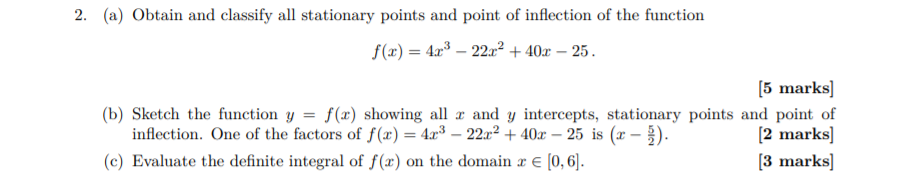2. (a) Obtain and classify all stationary points and point of inflection of the function f(x) = 4x3 – 22x2 + 40x – 25. [5 marks) (b) Sketch the function y = f(x) showing all x and y intercepts, stationary points and point of inflection. One of the factors of f(x) = 423 – 22cr2 + 40x – 25 is (r – ). [2 marks] (c) Evaluate the definite integral of f(c) on the domain 2 € (0,6]. [3 marks)

• ### Show ALL work to receive rating. Thanks! 1. Let f(x) = -4x^3+6x^2 a) Where is f(x)...

Show ALL work to receive rating. Thanks! 1. Let f(x) = -4x^3+6x^2 a) Where is f(x) increasing/decreasing? Make a sign chart. b) Classify the critical points as local max, local min, or neither. c) Where is f(x) concave up/concave down? Does it have any points of inflection? d) Use the information above to sketch the curve. Note that f(1/2) = 1. Be sure your graph includes the x and y intercepts if they exist.

• ### Sketch the graph of the function f(x) - (2-6)(x+3) 9(2+2) A sketch need not be exact...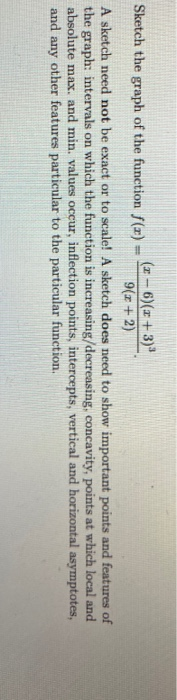Sketch the graph of the function f(x) - (2-6)(x+3) 9(2+2) A sketch need not be exact or to scale! A sketch does need to show important points and features of the graph: intervals on which the function is increasing/decreasing, concavity, points at which local and absolute max, and min. values occur, inflection points, intercepts, vertical and horizontal asymptotes, and any other features particular to the particular function,

• ### 6. (10 pts) Use calculus to sketch the graph of f(x) = 6.5-3x Show clearly the...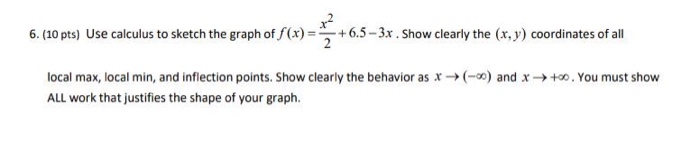6. (10 pts) Use calculus to sketch the graph of f(x) = 6.5-3x Show clearly the (x, y) coordinates of all (-) and x +0. You must show local max, local min, and inflection points. Show clearly the behavior as x ALL work that justifies the shape of your graph.

• ### 2.c 7. Sketch the function f(x) = = 5 and state the following (if any). Round...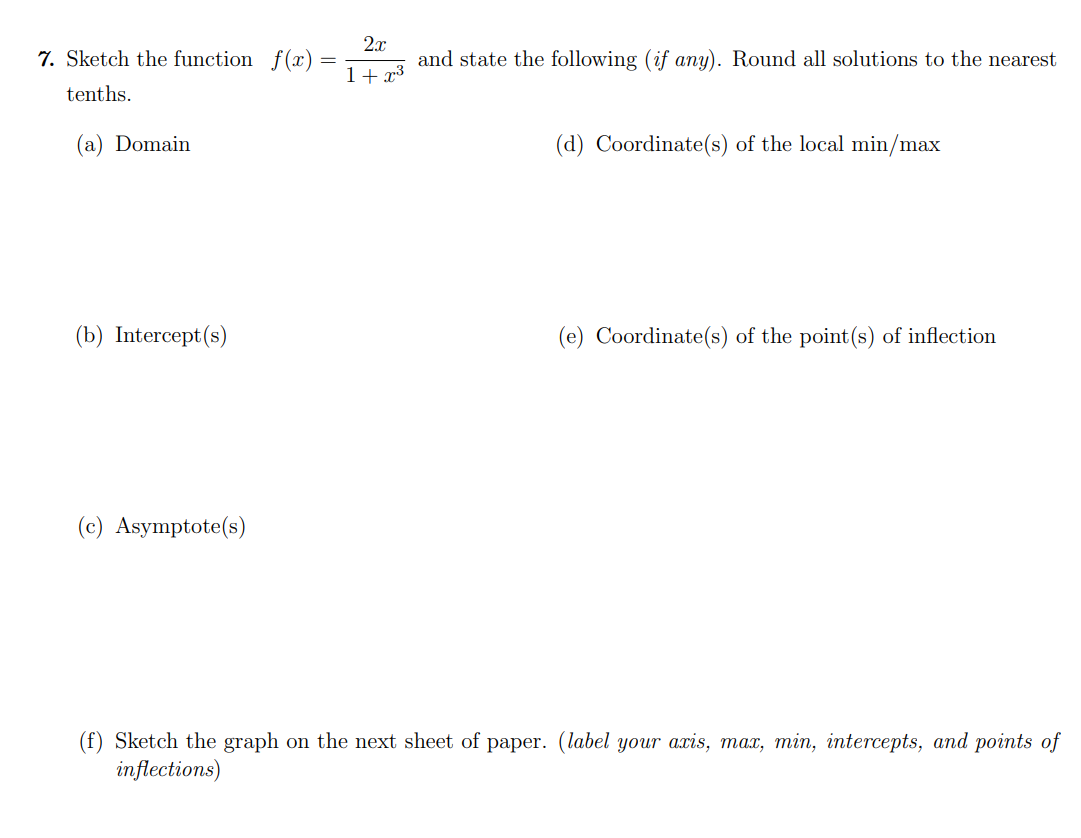2.c 7. Sketch the function f(x) = = 5 and state the following (if any). Round all solutions to the nearest 1+73 and state tenths. (a) Domain (d) Coordinate(s) of the local min/max (b) Intercept(s) (e) Coordinate(s) of the point(s) of inflection (c) Asymptote(s) (f) Sketch the graph on the next sheet of paper. (label your acis, mat, min, intercepts, and points of inflections)

• ### S o mo (StS 4. (20 pts) Analyze the graph of f(x) = 2x + 3x2...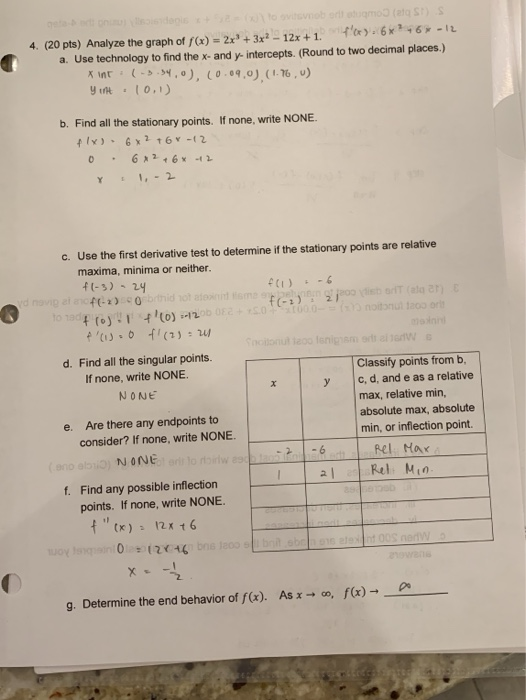S o mo (StS 4. (20 pts) Analyze the graph of f(x) = 2x + 3x2 - 12x +1. fo.6x246-12 a. Use technology to find the x-and y-intercepts. (Round to two decimal places.) x Int (-3.34,0), (0.09.0) (1.76, u) yint 10.1) b. Find all the stationary points. If none, write NONE. +x) - 6x2 +6 Y-12 0.6*26* 2 8 1 -2 C. Use the first derivative test to determine if the stationary points are relative maxima, minima or neither. f(-3)...

• ### I want all the working, Thankyou 1. Investigate the function based on the properties below. Then...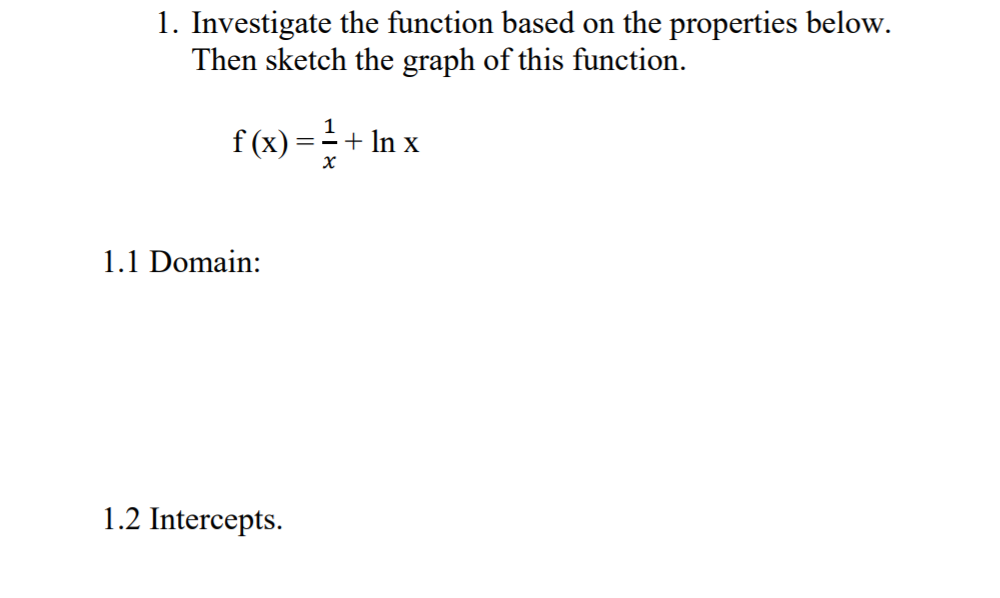I want all the working, Thankyou 1. Investigate the function based on the properties below. Then sketch the graph of this function. f(x)=+ In x 1.1 Domain: 1.2 Intercepts. 1.3 Symmetry. 1.4 Asymptotes. 1.5 Intervals where f(x) increasing/decreasing 1.6 Critical #. 1.7 Local max/min 1.8 Concavity 1.9 Inflection Points

• ### i need help with c, d, and e 3. Use the following steps to sketch the...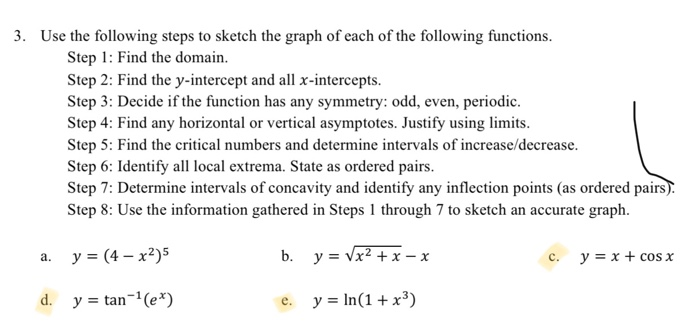i need help with c, d, and e 3. Use the following steps to sketch the graph of each of the following functions. Step 1: Find the domain. Step 2: Find the y-intercept and all x-intercepts. Step 3: Decide if the function has any symmetry: odd, even, periodic. Step 4: Find any horizontal or vertical asymptotes. Justify using limits. Step 5: Find the critical numbers and determine intervals of increase/decrease. Step 6: Identify all local extrema. State as ordered pairs....

• ### please solve b and c 3. Use the following steps to sketch the graph of each...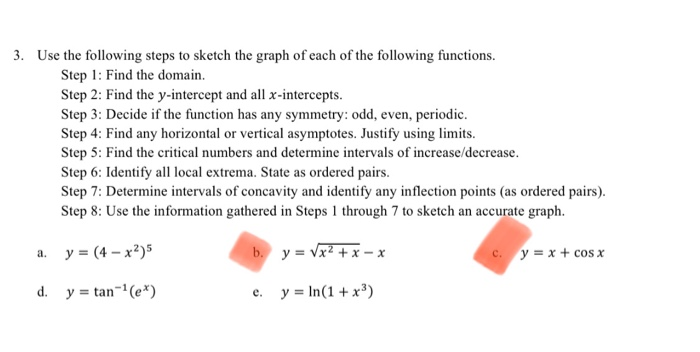please solve b and c 3. Use the following steps to sketch the graph of each of the following functions. Step 1: Find the domain. Step 2: Find the y-intercept and all x-intercepts. Step 3: Decide if the function has any symmetry: odd, even, periodic. Step 4: Find any horizontal or vertical asymptotes. Justify using limits. Step 5: Find the critical numbers and determine intervals of increase/decrease. Step 6: Identify all local extrema. State as ordered pairs. Step 7: Determine...

• ### Use the steps below to sketch the graph y = x^2 - 7x - 18. Required...

Use the steps below to sketch the graph y = x^2 - 7x - 18. Required points are the x intercepts and the max and mix of the graph 1. Determine the domain of f. 2. Find the x- and y-intercepts of f.† 3. Determine the behavior of f for large absolute values of x. 4. Find all horizontal and vertical asymptotes of the graph of f. 5. Determine the intervals where f is increasing and where f is decreasing....